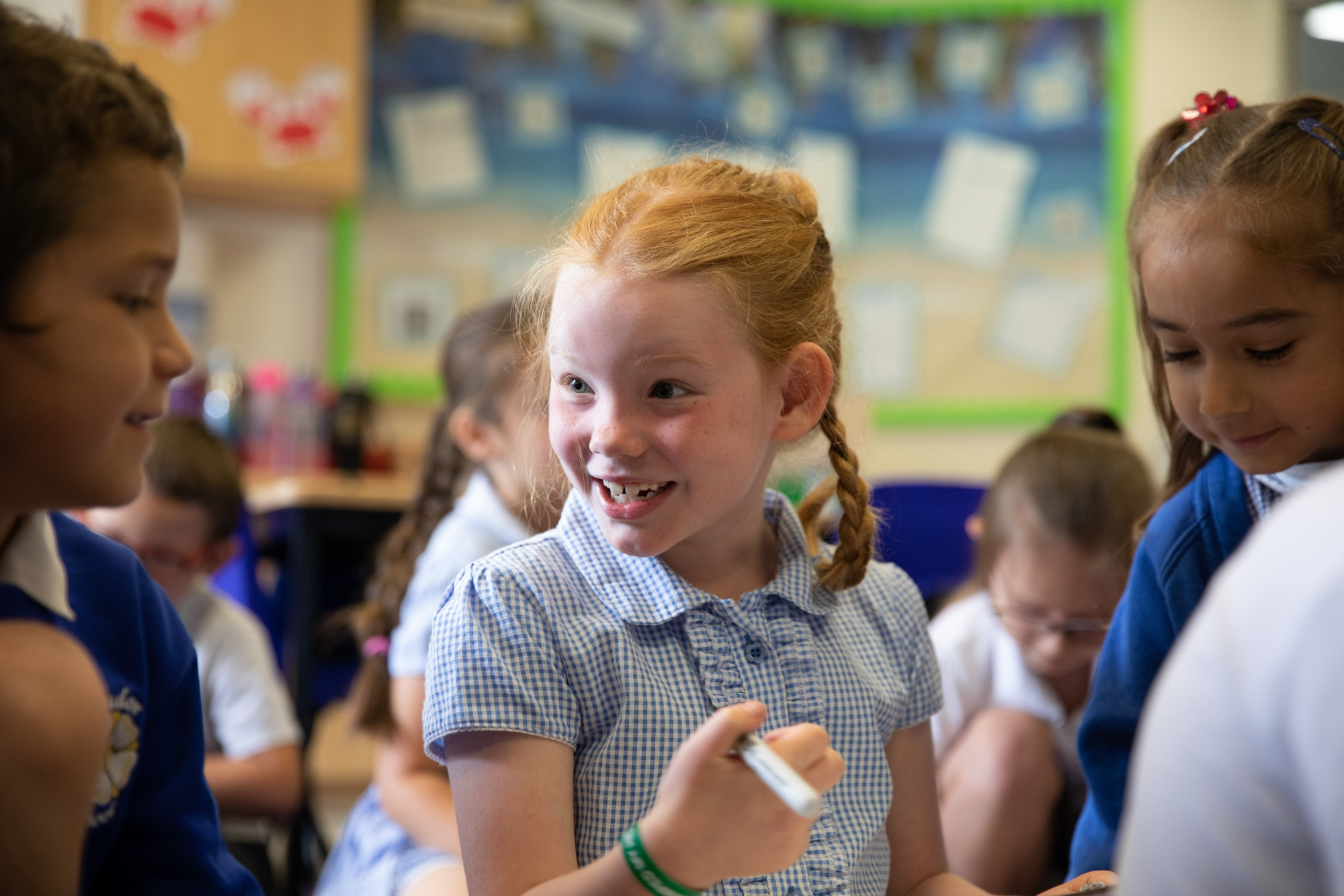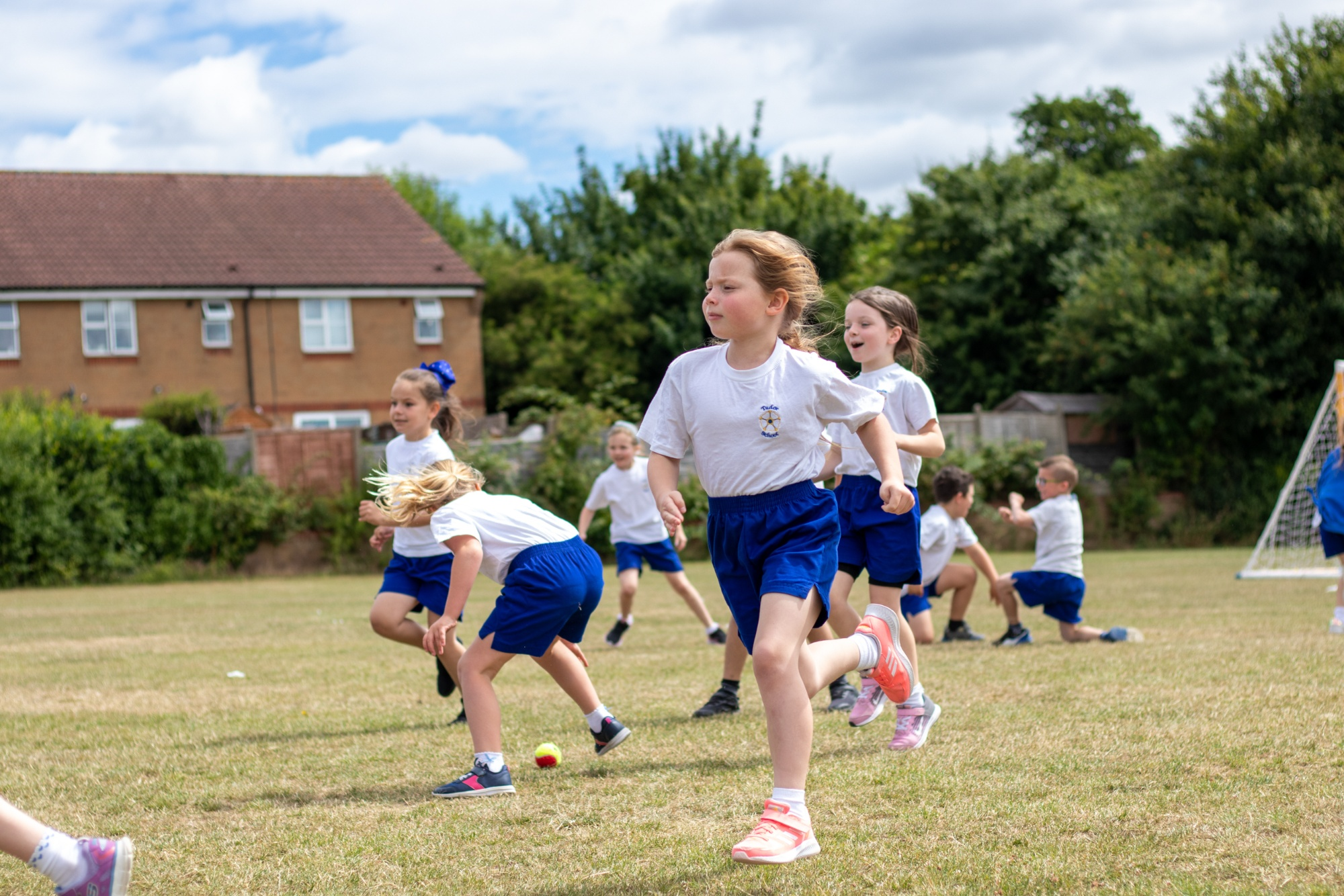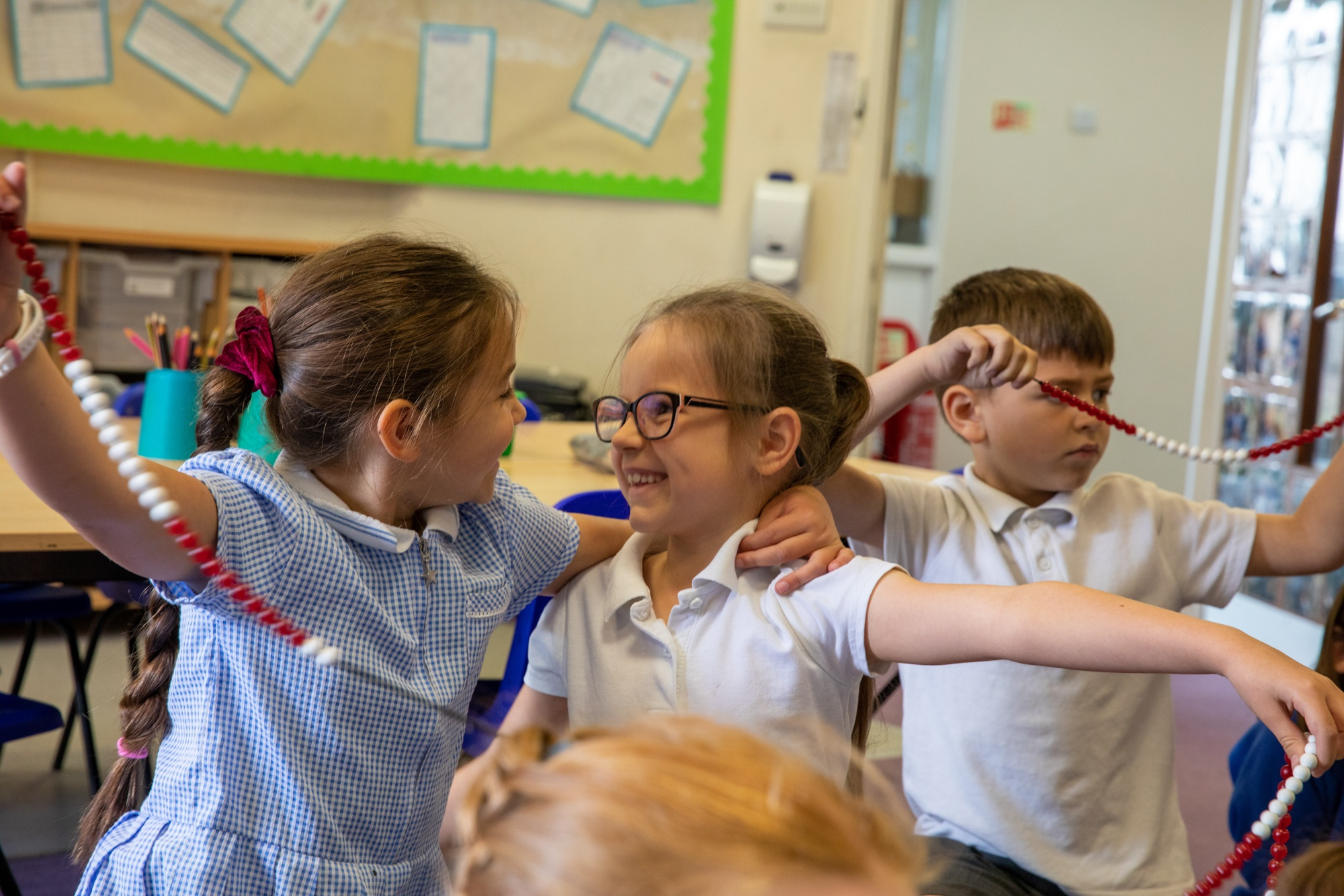Wednesday 1st April

Hi everyone,

Well done on your fantastic work today! It was so lovely to see you all on the Zoom story!

Here are the answers todays maths questions:

 * ** *** 65 + 10 = 75 33 + 12 = 45 43 + 23 = 66 154 + 224 = 378 123 + 129 = 252 216 + 135 = 351 676 + 199 = 877 348 + 396 = 744 432 + 298 = 730